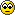## Understanding Fractions VisuallyThis is my first post about visual maths fractions and I wish it to be brief and to the point.

## What is a fraction?

Fraction is a part or parts of a whole.That is, only one part, piece, share, slice… or more parts/pieces/shares/slices of an entire thing.In short, a fraction can be from a very tiny part to a huge chunk or many, many parts of the whole thing – and anything in between.

## Why bother?

What is the point of understanding or learning fractions?
It is boring anyway, according to children!
Well, it turns out that people use fractions, in one form or another, in everyday life just like basic arithmetic.

## How to represent a fraction

A fraction is shown using a fraction line that looks like a long, horizontal dash with a pair of numbers, one on either side.The top number, above the fraction line, is called the numerator.
It shows how many parts out of a whole.
In our simple example, 1 indicates one part out of 2 parts.

The bottom number, below the fraction line, is the denominator.
It means the total parts, how many parts are there altogether, to make a whole.
In this case, the whole shape consists of just two parts – one shaded (red) and one unshaded (clear).
The fraction line, between the numerator and denominator, is a division operator just like a ÷ sign.

## Fractions Family

Fractions, decimal fractions, percentages and ratios are all the same thing, simply expressed differently.
That’s, the very same numbers or values but said, written or presented in different ways. For instance;

• A half or one-half equals ½, 0.5, 50% and 1:1 (equal parts or even chance).
• A quarter or one-quarter equals ¼ , 0.25, 25% and 1:3.

## Basic types of fractions

A fraction can be classified as a proper fraction, top-heavy fraction or mixed fraction.

I will try to use simple and similar examples for maximum clarification and consistency.

### Proper Fractions

This is when the top number (numerator) is less than the bottom number (denominator), resulting a decimal fraction or value that is less than one whole or 1.

For instance, ½ = 0.5 , ¼ = 0.25 and ¾ = 0.75.A proper fraction is also called a common fraction.

### Top-heavy Fractions

As the name suggests, the top number (numerator) is heavier or bigger than the bottom number (denominator). The resultant decimal fraction or value is bigger than one whole (1).

Examples, 3/2 = 1.5, 5/4 = 1.25 and 7/4 = 1.75.A top-heavy fraction is also called an improper fraction; i.e the opposite of a proper fraction for not having a numerator that’s less than the denominator.

### Mixed Fractions

As the name implies, this is a ‘mix’ of whole numbers and fractions.

For example, 1½ = 1.5, 1¼ = 1.25 and 1¾ = 1.75.Appropriately, a mixed fraction is also called a mixed number!

### Top-heavy Fractions to Mixed Fractions

A top-heavy fraction (an improper fraction) can be converted to a mixed fraction.

#### Step-by-step examples

1) Two children share three bars of chocolate .First, they each get one.Two bars gone. One red left.

Then, they share the red bar, to get a half each.In total, each child will have one-and-half bars.2) Four children share five bars of chocolate.First, every child gets one.Four bars taken. One green remaining.

Next, they share the green bar and get a quarter each.Therefore, every child will receive a total of one and a quarter bars.3) Four children share seven bars of chocolate.First, every child gets one bar.Four bars gone. Three blue left.

Now, let’s divide each blue bar into quarters to share for four children.The children share every blue bar to get a quarter from each whole bar.Hence three quarters, 3 x ¼. Or three-quarters of a whole, ¾.Finally, the share of each child adds up to one and three-quarters.That is how top-heavy fractions change to mixed fractions.

### Mixed Fractions to Top-heavy Fractions

A mixed fraction can be converted back to a top-heavy fraction.It is the opposite (reverse or inverse operation) of previous examples.

So, let’s gather our children to add up how much chocolate each group got!

#### Step-by-step examples

a) The first two children have one and a half bars each.That makes three whole bars shared by two children or 3/2.b) The next group of four children have one and a quarter bars each.Every two of them will have two and a half bars.Therefore, a group of four children will have twice of that of two children!
That is, double. Then double again.The result is five bars shared by four children or 5/4.

c) The last four children have one and three-quarters each.Now, every pair of children will have three and a half bars each.Finally, this group of four will share seven bars of chocolate or 7/4.### Tip!

Doubling a number, then doubling it again is the quickest and safest way if someone is struggling with their 4 times table.
This technique also works in reverse for divide by 4; by halving a number, then halving it again.

Someone still has problems with doubling and halving?
Well, then they are in trouble!(Seriously, especially at small numbers.)

That’s it for this post of Understanding Fractions Visually.

 Eng S Jama is an experienced electronics engineer turned an educational tutor and a self-published author.

Fractions Visuallis for children who think fractions are no fun and grown-ups who have never found the best visual resources to master basic maths fractions.

Ages 5-11, Year groups 1-6 and Key Stages 1-2.
Or internationally equivalents in primary curriculum (infants and junior schools).

1) Colouring/shading fraction shapes and tracing shapes, images & text2) Illustrated, visual fractionsor(Also, available as a workbook, colour workbook and colour e-books)
.

3) How to add visual fractionsor(Also, available as a workbook and colour workbook)

Series 1

Series 2

Series 3: Coming soon!